# Algebra II : Radicals as Exponents

## Example Questions

### Example Question #72 : Understanding Radicals

Rewrite the following radical as an exponent: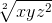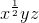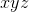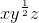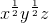Explanation:

In order to rewrite a radical as an exponent, the number in the radical that indicates the root, gets written as a fractional exponent. Distribute the exponent to all terms by multiplying it by the exponents of each term as shown below: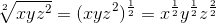From this point simplify the exponents accordingly: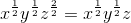### Example Question #73 : Understanding Radicals

Rewrite the following radical as an exponent: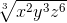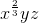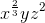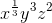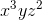Explanation:

In order to rewrite a radical as an exponent, the number in the radical that indicates the root, gets written as a fractional exponent. Distribute the exponent to all terms by multiplying it by the exponents of each term as shown below: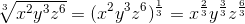From this point simplify the exponents accordingly: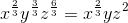### Example Question #74 : Understanding Radicals

Rewrite the following radical as an exponent: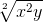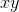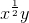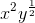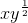Explanation:

In order to rewrite a radical as an exponent, the number in the radical that indicates the root, gets written as a fractional exponent. Distribute the exponent to both terms by multiplying it by the exponents of each term as shown below: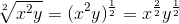From this point simplify the exponents accordingly: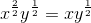### Example Question #75 : Understanding Radicals

Rewrite the following radical as an exponent: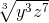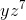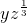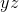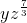Explanation:

In order to rewrite a radical as an exponent, the number in the radical that indicates the root, gets written as a fractional exponent. Distribute the exponent to both terms by multiplying it by the exponents of each term as shown below: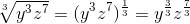From this point simplify the exponents accordingly: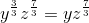### Example Question #76 : Understanding Radicals

Rewrite the following radical as an exponent: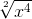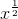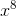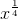Explanation:

In order to rewrite a radical as an exponent, the number in the radical that indicates the root, gets written as a fractional exponent. Distribute the exponent to both terms by multiplying it by the exponents of each term as shown below: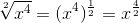From this point simplify the exponents accordingly: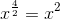### Example Question #77 : Understanding Radicals

Evaluate: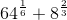Explanation:

In order to add the two terms, we must first find the values of each term in the expression.

Rewrite the fractional exponents as an expression of a square root.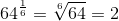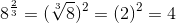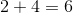The answer is:### Example Question #78 : Understanding Radicals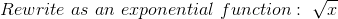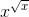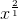Explanation:

Fractional exponents have the power as the numerator and the root as the denominator.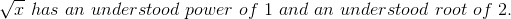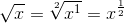### Example Question #21 : Radicals As Exponents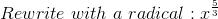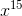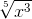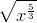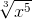Explanation:

Fractional exponents have the power as the numerator and the root as the denominator.

In this case, the power is 5, and the root is 3.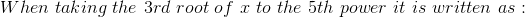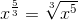### Example Question #80 : Understanding Radicals

Solve: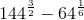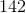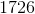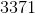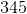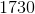Explanation:

The numbers with the fractional exponents can be rewritten as radicals.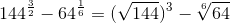Simplify both radicals.  The sixth root of 64 is two.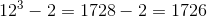The answer is:### Example Question #81 : Understanding Radicals

Solve: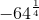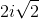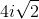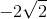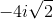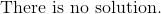Explanation:

This can be rewritten as the fourth root.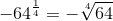Rewrite the inner quantity with factors of numbers to the fourth root.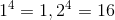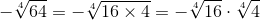Simplify both terms.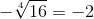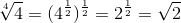The answer is:### All Algebra II Resources